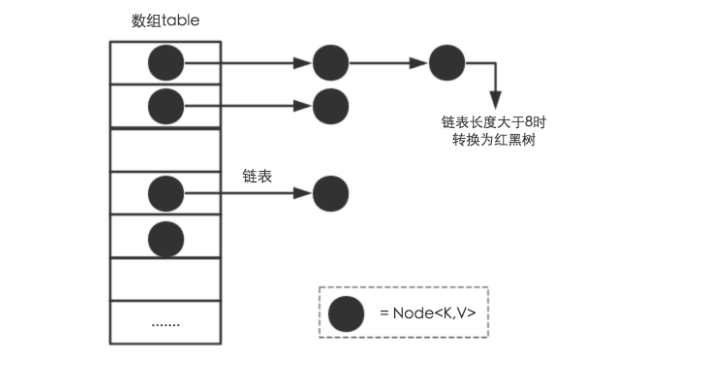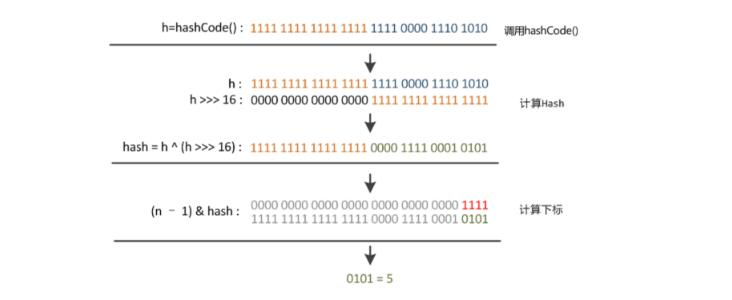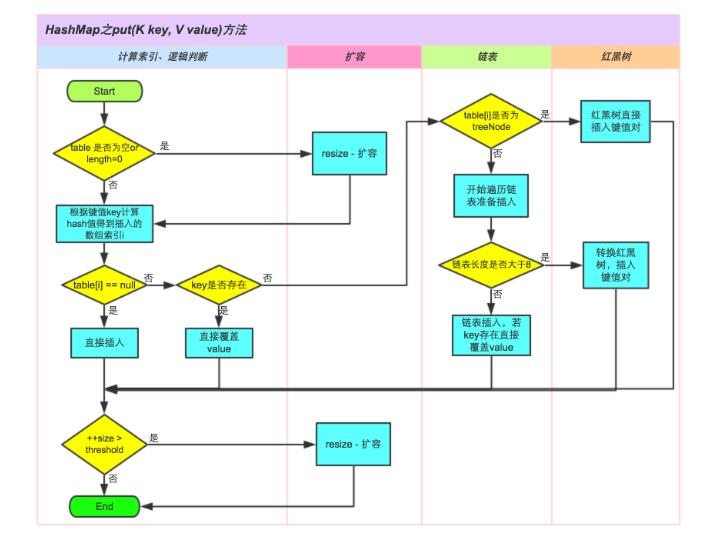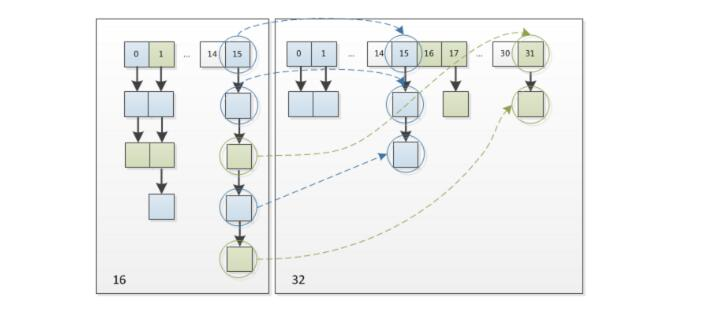## java集合框架之HashMap

starlin 475 2018-05-03

### HashMap概述

HashMap是我们使用最多的的Collection，它是基于哈希表的Map接口的实现，以key-value的形式存储，系统会根据hash算法来计算key-value的存储位置。

HashMap最多只允许一条记录的键为null，允许多条记录的值为null。

HashMap并不是一个线程安全的类，如果需要进行多线程的访问，可以考虑一下方式：

1. Map map = Collections.synchronizedMap(new HashMap(...))
2. 使用HashTable
3. 使用ConcurrentHashMap(推荐使用)

### HashMap源码解析

#### HashMap定义

HashMap实现了Map接口，继承AbstractMap，其中Map接口定义了键映射到值的规则，而AbstractMap类提供Map 接口的骨干实现，其接口实现如下：

public class HashMap<K,V> extends AbstractMap<K,V>
implements Map<K,V>, Cloneable, Serializable


#### HashMap构造函数

HashMap提供了4个构造函数：

    /**
* Constructs an empty <tt>HashMap</tt> with the default initial capacity
* (16) and the default load factor (0.75).
*/
//构造一个具有默认初始容量 (16) 和默认加载因子 (0.75) 的空 HashMap
public HashMap() {
}

/**
* Constructs an empty <tt>HashMap</tt> with the specified initial
* capacity and the default load factor (0.75).
*
* @param  initialCapacity the initial capacity.
* @throws IllegalArgumentException if the initial capacity is negative.
*/
//构造一个带指定初始容量和默认加载因子 (0.75) 的空 HashMap
public HashMap(int initialCapacity) {
}

/**
* Constructs an empty <tt>HashMap</tt> with the specified initial
*
* @param  initialCapacity the initial capacity
* @throws IllegalArgumentException if the initial capacity is negative
*         or the load factor is nonpositive
*/
//构造一个带指定初始容量和指定加载因子的空 HashMap
public HashMap(int initialCapacity, float loadFactor) {
if (initialCapacity < 0)
throw new IllegalArgumentException("Illegal initial capacity: " +
initialCapacity);
if (initialCapacity > MAXIMUM_CAPACITY)
initialCapacity = MAXIMUM_CAPACITY;
throw new IllegalArgumentException("Illegal load factor: " +
this.threshold = tableSizeFor(initialCapacity);
}

/**
* Constructs a new <tt>HashMap</tt> with the same mappings as the
* specified <tt>Map</tt>.  The <tt>HashMap</tt> is created with
* default load factor (0.75) and an initial capacity sufficient to
* hold the mappings in the specified <tt>Map</tt>.
*
* @param   m the map whose mappings are to be placed in this map
* @throws  NullPointerException if the specified map is null
*/
//用m的元素初始化散列映射
public HashMap(Map<? extends K, ? extends V> m) {
putMapEntries(m, false);
}


#### HashMap存储机制HashMap就是使用哈希表来存储的。哈希表为解决冲突，可以采用开放地址法和链地址法等来解决问题，Java中HashMap采用了链地址法。链地址法，简单来说，就是数组加链表的结合。在每个数组元素上都一个链表结构，当数据被Hash后，得到数组下标，把数据放在对应下标元素的链表上，例如执行下面代码：

map.put("starlin","111")

int threshold; // 所能容纳的key-value对极限
int modCount;
int size;

size这个字段其实很好理解，就是HashMap中实际存在的键值对数量。注意和table的长度length、容纳最大键值对数量threshold的区别。而modCount字段主要用来记录HashMap内部结构发生变化的次数，主要用于迭代的快速失败。强调一点，内部结构发生变化指的是结构发生变化，例如put新键值对，但是某个key对应的value值被覆盖不属于结构变化。

#### HashMap的数据结构

##### 1. HashMap中的属性
public class HashMap<k,v> extends AbstractMap<k,v> implements Map<k,v>, Cloneable, Serializable {
private static final long serialVersionUID = 362498820763181265L;
static final int DEFAULT_INITIAL_CAPACITY = 1 << 4; // aka 16
static final int MAXIMUM_CAPACITY = 1 << 30;//最大容量
static final float DEFAULT_LOAD_FACTOR = 0.75f;//填充比
static final int TREEIFY_THRESHOLD = 8;
static final int UNTREEIFY_THRESHOLD = 6;
static final int MIN_TREEIFY_CAPACITY = 64;
transient Node<k,v>[] table;//存储元素的数组
transient Set<map.entry<k,v>> entrySet;
transient int size;//存放元素的个数
transient int modCount;//被修改的次数fast-fail机制
int threshold;//临界值 当实际大小(容量*填充比)超过临界值时，会进行扩容
//（......后面略）

##### 2. 数组元素Node<K,V>实现了Entry接口

Node是HashMap的一个内部类，实现了Map.Entry接口，本质是就是一个映射(键值对)。上图中的每个黑色圆点就是一个Node对象。

    /**
* Basic hash bin node, used for most entries.  (See below for
* TreeNode subclass, and in LinkedHashMap for its Entry subclass.)
*/
static class Node<K,V> implements Map.Entry<K,V> {
//用来定位数组索引位置
final int hash;
final K key;
V value;
//链表的下一个node
Node<K,V> next;
//构造函数Hash值 键 值 下一个节点
Node(int hash, K key, V value, Node<K,V> next) {
this.hash = hash;
this.key = key;
this.value = value;
this.next = next;
}

public final K getKey()        { return key; }
public final V getValue()      { return value; }
public final String toString() { return key + "=" + value; }

public final int hashCode() {
return Objects.hashCode(key) ^ Objects.hashCode(value);
}

public final V setValue(V newValue) {
V oldValue = value;
value = newValue;
return oldValue;
}

//判断两个node是否相等,若key和value都相等，返回true。可以与自身比较为true
public final boolean equals(Object o) {
if (o == this)
return true;
if (o instanceof Map.Entry) {
Map.Entry<?,?> e = (Map.Entry<?,?>)o;
if (Objects.equals(key, e.getKey()) &&
Objects.equals(value, e.getValue()))
return true;
}
return false;
}
}

##### 3. 红黑树
    /**
* Entry for Tree bins. Extends LinkedHashMap.Entry (which in turn
* extends Node) so can be used as extension of either regular or
*/
static final class TreeNode<K,V> extends LinkedHashMap.Entry<K,V> {
TreeNode<K,V> parent;  // 父节点
TreeNode<K,V> left;    //左节点
TreeNode<K,V> right;    //右节点
TreeNode<K,V> prev;    // needed to unlink next upon deletion
boolean red;     //颜色属性
TreeNode(int hash, K key, V val, Node<K,V> next) {
super(hash, key, val, next);
}

/**
* Returns root of tree containing this node.
*/
//返回当前节点的根节点
final TreeNode<K,V> root() {
for (TreeNode<K,V> r = this, p;;) {
if ((p = r.parent) == null)
return r;
r = p;
}
}
//后面省略.....
}

##### 4. HashMap的存取
###### 4.1 确定哈希桶数组索引位置JDK7/8区别

static final int hash(Object key) {   //jdk1.8
int h;
// h = key.hashCode() 为第一步 取hashCode值
// h ^ (h >>> 16)  为第二步 高位参与运算
return (key == null) ? 0 : (h = key.hashCode()) ^ (h >>> 16);
}


static int indexFor(int h, int length) {  //jdk1.7的源码，jdk1.8没有这个方法，但是实现原理一样的
return h & (length-1);  //第三步 取模运算
}###### 4.2 put方法JDK8

HashMap的put方法执行过程可以通过下图来理解JDK1.8HashMap的put方法源码如下:

    public V put(K key, V value) {
return putVal(hash(key), key, value, false, true);
}

/**
* Implements Map.put and related methods
* @param hash hash for key
* @param key the key
* @param value the value to put
* @param onlyIfAbsent if true, don't change existing value
* @param evict if false, the table is in creation mode.
* @return previous value, or null if none
*/
final V putVal(int hash, K key, V value, boolean onlyIfAbsent,
boolean evict) {
Node<K,V>[] tab; Node<K,V> p; int n, i;
//第一次 put 值的时候，会触发下面的 resize()，类似 java7 的第一次 put 也要初始化数组长度
//第一次 resize 和后续的扩容有些不一样，因为这次是数组从 null 初始化到默认的 16 或自定义
//的初始容量
if ((tab = table) == null || (n = tab.length) == 0)
n = (tab = resize()).length;
//如果table的在（n-1）&hash的值是空，就新建一个节点插入在该位置
if ((p = tab[i = (n - 1) & hash]) == null)
tab[i] = newNode(hash, key, value, null);
else {//该位置有数据，表示有冲突，处理冲突
Node<K,V> e; K k;
//节点key存在，直接覆盖value
if (p.hash == hash &&
((k = p.key) == key || (key != null && key.equals(k))))
e = p;
//判断该链为红黑树 ，这里不展开了
else if (p instanceof TreeNode)
e = ((TreeNode<K,V>)p).putTreeVal(this, tab, hash, key, value);
else {//到这里，说明数组该位置上是一个链表
for (int binCount = 0; ; ++binCount) {
//插入到链表的最后面(Java7 是插入到链表的最前面)
if ((e = p.next) == null) {
p.next = newNode(hash, key, value, null);
//TREEIFY_THRESHOLD 为 8，所以，如果新插入的值是链表中的第 8 个
//会触发下面的 treeifyBin，也就是将链表转换为红黑树
//**注意treeifyBin方法里面还有一个判断，也就是hash表的的长度大于MIN_TREEIFY_CAPACITY（64），才会转换，否则只扩容**
if (binCount >= TREEIFY_THRESHOLD - 1) // -1 for 1st
treeifyBin(tab, hash);
break;
}
//如果在该链表中找到了"相等"的 key(== 或 equals)
//key已经存在直接覆盖value
if (e.hash == hash &&
((k = e.key) == key || (key != null && key.equals(k))))
break;
p = e;
}
}
//e!=null 说明存在旧值的key与要插入的key"相等"
if (e != null) { // existing mapping for key 表示key的Value存在
V oldValue = e.value;
if (!onlyIfAbsent || oldValue == null)
e.value = value;
afterNodeAccess(e);
//返回存在的value值
return oldValue;
}
}
++modCount;
//超过最大容量 就扩容
if (++size > threshold)
resize();
afterNodeInsertion(evict);
return null;
}


treeifyBin方法：

//将桶内所有的 链表节点 替换成 红黑树节点
final void treeifyBin(Node<K,V>[] tab, int hash) {
int n, index; Node<K,V> e;
//如果当前哈希表为空，或者哈希表中元素的个数小于 进行树形化的阈值(默认为 64)，就去新建/扩容
if (tab == null || (n = tab.length) < MIN_TREEIFY_CAPACITY)
resize();
else if ((e = tab[index = (n - 1) & hash]) != null) {
//如果哈希表中的元素个数超过了 树形化阈值，进行树形化
// e 是哈希表中指定位置桶里的链表节点，从第一个开始
TreeNode<K,V> hd = null, tl = null; //红黑树的头、尾节点
do {
//新建一个树形节点，内容和当前链表节点 e 一致
TreeNode<K,V> p = replacementTreeNode(e, null);
if (tl == null) //确定树头节点
hd = p;
else {
p.prev = tl;
tl.next = p;
}
tl = p;
} while ((e = e.next) != null);
//让桶的第一个元素指向新建的红黑树头结点，以后这个桶里的元素就是红黑树而不是链表了
if ((tab[index] = hd) != null)
hd.treeify(tab);
}
}

TreeNode<K,V> replacementTreeNode(Node<K,V> p, Node<K,V> next) {
return new TreeNode<>(p.hash, p.key, p.value, next);



1，判断键值对数组tab[]是否为空或为null，否则以默认大小resize()；
2，根据键值key计算hash值得到插入的数组索引i，如果tab[i]==null，直接新建节点添加，否则转入3
3，判断当前数组中处理hash冲突的方式为链表还是红黑树(check第一个节点类型即可),分别处理

###### 4.3 put方法JDK7

JDK1.7HashMap的put方法源码如下:

    public V put(K key, V value) {
// 当插入第一个元素的时候，需要先初始化数组大小
if (table == EMPTY_TABLE) {
inflateTable(threshold);
}
// 如果 key 为 null，感兴趣的可以往里看，最终会将这个 entry 放到 table 中
if (key == null)
return putForNullKey(value);
// 1. 求 key 的 hash 值
int hash = hash(key);
// 2. 找到对应的数组下标
int i = indexFor(hash, table.length);
// 3. 遍历一下对应下标处的链表，看是否有重复的 key 已经存在，
//    如果有，直接覆盖，put 方法返回旧值就结束了
for (Entry<K,V> e = table[i]; e != null; e = e.next) {
Object k;
if (e.hash == hash && ((k = e.key) == key || key.equals(k))) {
V oldValue = e.value;
e.value = value;
e.recordAccess(this);
return oldValue;
}
}

modCount++;
// 4. 不存在重复的 key，将此 entry 添加到链表中
return null;
}


    private void inflateTable(int toSize) {
// 保证数组大小一定是 2 的 n 次方。
// 比如这样初始化：new HashMap(20)，那么处理成初始数组大小是 32
int capacity = roundUpToPowerOf2(toSize);
threshold = (int) Math.min(capacity * loadFactor, MAXIMUM_CAPACITY + 1);
// 算是初始化数组吧
table = new Entry[capacity];
initHashSeedAsNeeded(capacity); //ignore
}


    void addEntry(int hash, K key, V value, int bucketIndex) {
// 如果当前 HashMap 大小已经达到了阈值，并且新值要插入的数组位置已经有元素了，那么要扩容
if ((size >= threshold) && (null != table[bucketIndex])) {
// 扩容，后面会介绍一下
resize(2 * table.length);
// 扩容以后，重新计算 hash 值
hash = (null != key) ? hash(key) : 0;
// 重新计算扩容后的新的下标
bucketIndex = indexFor(hash, table.length);
}
// 往下看
createEntry(hash, key, value, bucketIndex);
}

// 这个很简单，其实就是将新值放到链表的表头，然后 size++
void createEntry(int hash, K key, V value, int bucketIndex) {
Entry<K,V> e = table[bucketIndex];
table[bucketIndex] = new Entry<>(hash, key, value, e);
size++;
}

###### 4.4 扩容机制JDK8

JDK1.8中的resize源码如下：

/**
* Initializes or doubles table size.  If null, allocates in
* accord with initial capacity target held in field threshold.
* Otherwise, because we are using power-of-two expansion, the
* elements from each bin must either stay at same index, or move
* with a power of two offset in the new table.
*
* @return the table
*/
final Node<K,V>[] resize() {
Node<K,V>[] oldTab = table;
int oldCap = (oldTab == null) ? 0 : oldTab.length;
int oldThr = threshold;
int newCap, newThr = 0;
//如果旧表的长度不是空
if (oldCap > 0) {
//超过最大值就不再扩充了
if (oldCap >= MAXIMUM_CAPACITY) {
threshold = Integer.MAX_VALUE;
return oldTab;
}//没有超过最大值，就扩充为原来2倍
else if ((newCap = oldCap << 1) < MAXIMUM_CAPACITY &&
oldCap >= DEFAULT_INITIAL_CAPACITY)
newThr = oldThr << 1; // double threshold
}
//如果旧表的长度的是0，就是说第一次初始化表
else if (oldThr > 0) // initial capacity was placed in threshold
newCap = oldThr;
else {               // zero initial threshold signifies using defaults
newCap = DEFAULT_INITIAL_CAPACITY;
}
//计算新的resize上限
if (newThr == 0) {
float ft = (float)newCap * loadFactor;//新表长度乘以加载因子
newThr = (newCap < MAXIMUM_CAPACITY && ft < (float)MAXIMUM_CAPACITY ?
(int)ft : Integer.MAX_VALUE);
}
threshold = newThr;
@SuppressWarnings({"rawtypes","unchecked"})
// 用新的数组大小初始化新的数组
Node<K,V>[] newTab = (Node<K,V>[])new Node[newCap];
table = newTab;//把新表赋值给table
if (oldTab != null) {//开始遍历原数组，进行数据迁移
//把每个bucket都移动到新的buckets中
for (int j = 0; j < oldCap; ++j) {
Node<K,V> e;
if ((e = oldTab[j]) != null) {
oldTab[j] = null;
//如果该数组位置上只有单个元素，那就简单了，简单迁移这个元素就可以了
//也就是说这个node没有链表，是单个元素，直接放在新表的e.hash & (newCap - 1)位置
if (e.next == null)
newTab[e.hash & (newCap - 1)] = e;
else if (e instanceof TreeNode)//如果是红黑树，就不具体说了
((TreeNode<K,V>)e).split(this, newTab, j, oldCap);
else { // preserve order
//这里是处理链表,链表优化重hash的代码块
//需要将此链表拆成两个链表，放到新的数组中，并且保留原来的先后顺序
Node<K,V> loHead = null, loTail = null;
Node<K,V> hiHead = null, hiTail = null;
Node<K,V> next;
do {
next = e.next;//记录下一个结点
// 原索引
if ((e.hash & oldCap) == 0) {
if (loTail == null)
else
loTail.next = e;
loTail = e;
}
// 原索引+oldCap
else {
if (hiTail == null)
else
hiTail.next = e;
hiTail = e;
}
} while ((e = next) != null);
// 原索引放到bucket里
if (loTail != null) {
loTail.next = null;
//第一条链表
}
// 原索引+oldCap放到bucket里
if (hiTail != null) {
hiTail.next = null;
//第二条链表的新的位置是 j + oldCap
}
}
}
}
}
return newTab;
}###### 4.5 扩容机制JDK7

JDK1.7的扩容机制源码如下：

    void resize(int newCapacity) {   //传入新的容量
Entry[] oldTable = table;    //引用扩容前的Entry数组
int oldCapacity = oldTable.length;
if (oldCapacity == MAXIMUM_CAPACITY) {  //扩容前的数组大小如果已经达到最大(2^30)了
threshold = Integer.MAX_VALUE; //修改阈值为int的最大值(2^31-1)，这样以后就不会扩容了
return;
}

Entry[] newTable = new Entry[newCapacity];  //初始化一个新的Entry数组
transfer(newTable);                         //！！将数据转移到新的Entry数组里
table = newTable;                           //HashMap的table属性引用新的Entry数组
}


transfer()方法将原有Entry数组的元素拷贝到新的Entry数组里，源码如下：

  void transfer(Entry[] newTable) {
Entry[] src = table;                   //src引用了旧的Entry数组
int newCapacity = newTable.length;
for (int j = 0; j < src.length; j++) { //遍历旧的Entry数组
Entry<K,V> e = src[j];             //取得旧Entry数组的每个元素
if (e != null) {
src[j] = null;//释放旧Entry数组的对象引用（for循环后，旧的Entry数组不再引用任何对象）
do {
Entry<K,V> next = e.next;
int i = indexFor(e.hash, newCapacity); //！！重新计算每个元素在数组中的位置
e.next = newTable[i]; //标记
newTable[i] = e;      //将元素放在数组上
e = next;             //访问下一个Entry链上的元素
} while (e != null);
}
}
}


newTable[i]的引用赋给了e.next，也就是使用了单链表的头插入方式，同一位置上新元素总会被放在链表的头部位置；这样先放在一个索引上的元素终会被放到Entry链的尾部(如果发生了hash冲突的话）

###### 4.6 get方法JDK8

1. 计算 key 的 hash 值，根据 hash 值找到对应数组下标
2. 判断数组该位置处的元素是否刚好就是我们要找的，如果不是，走第三步
3. 判断该元素类型是否是 TreeNode，如果是，用红黑树的方法取数据，如果不是，走第四步
4. 遍历链表，直到找到相等(==或equals)的 key

    public V get(Object key) {
Node<K,V> e;
return (e = getNode(hash(key), key)) == null ? null : e.value;
}

/**
* Implements Map.get and related methods
*/
final Node<K,V> getNode(int hash, Object key) {
Node<K,V>[] tab; Node<K,V> first, e; int n; K k;
if ((tab = table) != null && (n = tab.length) > 0 &&
(first = tab[(n - 1) & hash]) != null) {
//判断第一个节点是不是需要的
if (first.hash == hash && // always check first node
((k = first.key) == key || (key != null && key.equals(k))))
return first;
if ((e = first.next) != null) {
//判断是否是红黑树
if (first instanceof TreeNode)
return ((TreeNode<K,V>)first).getTreeNode(hash, key);
//链表遍历
do {
if (e.hash == hash &&
((k = e.key) == key || (key != null && key.equals(k))))
return e;
} while ((e = e.next) != null);
}
}
return null;
}

###### 4.7 get方法JDK7

JDK7的get过程大概分为以下几步：

1. 根据 key 计算 hash 值。
2. 找到相应的数组下标，hash & (length - 1)
3. 遍历该数组位置处的链表，直到找到相等(==或equals)的 key。

    public V get(Object key) {
// 之前说过，key 为 null 的话，会被放到 table，所以只要遍历下 table 处的链表就可以了
if (key == null)
return getForNullKey();
//
Entry<K,V> entry = getEntry(key);

return null == entry ? null : entry.getValue();
}


getEntry(key)方法：

    final Entry<K,V> getEntry(Object key) {
if (size == 0) {
return null;
}

int hash = (key == null) ? 0 : hash(key);
// 确定数组下标，然后从头开始遍历链表，直到找到为止
for (Entry<K,V> e = table[indexFor(hash, table.length)];
e != null;
e = e.next) {
Object k;
if (e.hash == hash &&
((k = e.key) == key || (key != null && key.equals(k))))
return e;
}
return null;
}